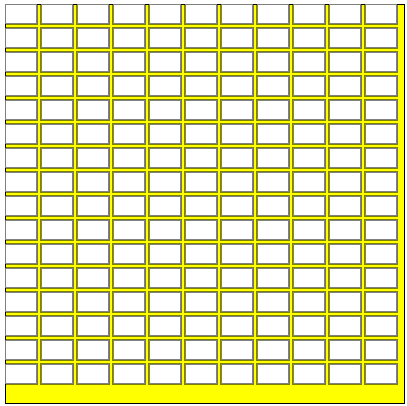Engineering ToolBox - Resources, Tools and Basic Information for Engineering and Design of Technical Applications!

# Smaller Rectangles within a Larger Rectangle

## The maximum number of smaller rectangles - or squares - within a larger rectangle (or square).

The calculator below can be used to estimate the maximum number of smaller rectangles - or squares - that may fit into a larger rectangle or square. The calculator can be used to calculate applicatons like

• the number of bricks in a wall
• the number of rectangular cuts possible from a steel or plywood plate

Input the large rectangle inside dimensions - and the outside dimensions of the smaller rectangles.

Default values are for 0.5 x 0.8 inch rectangle inside a 10 inch x 10 inch square. The calculator is generic and all units can be used - as long as the same units are used for all values.

large rectangle width (in, mm, m)

large rectangle height (in, mm, m)

small rectangles width (in, mm, m)

small rectangles height (in, mm, m)

space between rectangles (in, mm, m)

### Rectangular PatternNote! - the algorithm used for this tool is quite simple. Be aware that for some combinations of values more rectangles can be added by rearranging the layout.

## Related Topics

• ### Mathematics

Mathematical rules and laws - numbers, areas, volumes, exponents, trigonometric functions and more.

## Related Documents

• ### Area of Intersecting Circles

Calculate area of intersecting circles
• ### Area Units Converter

Convert between units of area.
• ### Bricks - Quantity and Mortar Consumption

Estimate required quantities of bricks and mortar.
• ### Centroids of Plane Areas

The controid of square, rectangle, circle, semi-circle and right-angled triangle.
• ### Circle - Equation

The equation for a circle
• ### Circle - the Chord Lengths when Divided in to Equal Segments

Calculate chord lengths when dividing the circumference of a circle into an equal number of segments.
• ### Circles - Circumferences and Areas

Circumferences and areas of circles with diameters in inches.
• ### Circles Outside a Circle

Calculate the numbers of circles on the outside of an inner circle - like the geometry of rollers on a shaft.
• ### Circles within a Rectangle - Calculator

Calculate the maximum number of circles within a rectangle - can be used to calculate the numbers of pipes or wires in a conduit or similar.
• ### Equal Areas - Circles vs. Squares

Radius and side lengths of equal areas, circles and squares.
• ### Fluid Flow - Equivalent Diameter

Convert rectangular and oval duct geometry to a equivalent circular diameter - online calculator with imperial and SI-units.
• ### Geometric Shapes - Areas

Areas, diagonals and more - of geometric figures like rectangles, triangles, trapezoids ..
• ### Masonry Blocks - Dimensions

Concrete Masonry Unit Blocks (CMU) - dimensions.
• ### Smaller Circles within a Large Circle - Calculator

Calculate the number of small circles that fits into an outer larger circle - ex. how many pipes or wires fits into a larger pipe or conduit.
• ### Squaring with Diagonal Measurements

A rectangle is square if the lengths of both diagonals are equal.

## Engineering ToolBox - SketchUp Extension - Online 3D modeling!

Add standard and customized parametric components - like flange beams, lumbers, piping, stairs and more - to your Sketchup model with the Engineering ToolBox - SketchUp Extension - enabled for use with older versions of the amazing SketchUp Make and the newer "up to date" SketchUp Pro . Add the Engineering ToolBox extension to your SketchUp Make/Pro from the Extension Warehouse !

We don't collect information from our users. More about

## Citation

• The Engineering ToolBox (2018). Smaller Rectangles within a Larger Rectangle. [online] Available at: https://www.engineeringtoolbox.com/smaller-rectangles-within-larger-rectangle-d_2111.html [Accessed Day Month Year].

Modify the access date according your visit.

9.19.12